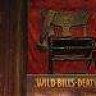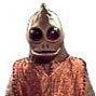M

#### mstram

##### Rock Star
Is this correct ?

Probability of being dealt any badugi (R1a R2b R3c R4d)

4/52 * 3/51 * 2/50 * 1/49

If that is correct, how would you calculate the probability for a particular hand? E.g. Aa 2b 3c 4d#### Dwilius

##### CardsChat Regular
odds of being dealt a nut badugi would be ((4 *4)/52) x ((3*3)/51) x ((2*2)/50) x (1/49). Oh, I guess you mean any badugi, all the way up to K? That would be ((12*3)/51) x ((11*2)/50) x (10/50)

...your numbers are the odds of an exact hand, suit matching the value.

Last edited:#### Exit141RTe1

##### Legend
I tried this game a month or so ago. What a headache I had when the game was over.

I will try it again but I have some reading to do and work out the odds listed above so I understand it.

M

#### mstram

##### Rock Star

We can calculate the number of badugis with high card N as ((N-1) choose 3) * 4!
(That is, we must choose 1 card of rank N, 3 different ranks from the
remaining N-1 ranks, and they may have any assignment of four different suits.)

As an almost "math illiterate", that's a pretty slick forumula !

I can understand the (N-1) choose 3), especially since it is "spelled out" in english, but I don't get the 4 factorial for the suits.

Can anyone explain why / how the 4 factorial relates to the suits ?

Pretend you are talking to a 6 year oldLast edited by a moderator:
O

#### only_bridge

##### Legend
The 4! comes from the 4 suits, its correct.
The first card can be chosen from 4 suits, the 2:nd from 3 suits, the 3:rd from 2, and the last from just one suit.
The multiply theroem then gives us 4x3x2x1=4!
The formula calculates the # of badugi hands exactly n high.
If you want to calculate the total # of badugi hands that are n high or better, then the formula would be:
(n choose 4)4!

Last edited:
M

#### mstram

##### Rock Star

If drawing 2 cards to an 8 or bettter, starting with two cards <=8.

So draw 1:

12 outs from remaining 48 cards = 33 %

Draw 2 : 10 outs from 46 cards = 27%

Draw 3: 8 outs from 44 cards = 22%

How do you calculate the total percentage for all three draws combined?

I.e Calculate the odds of hitting on three draws?

You can't just add those percentages can you ? ... giving 82% of hitting ?Mike

O

#### only_bridge

##### Legend
If drawing 2 cards to an 8 or bettter, starting with two cards <=8.

So draw 1:

12 outs from remaining 48 cards = 33 %

Draw 2 : 10 outs from 46 cards = 27%

Draw 3: 8 outs from 44 cards = 22%

How do you calculate the total percentage for all three draws combined?

I.e Calculate the odds of hitting on three draws?

You can't just add those percentages can you ? ... giving 82% of hitting ?Mike
I dont really understand what you want to calculate, but lets say you have 3 cards, 8 high, and you are drawing for an 8 high badugi.
Then the chance of hitting would be:
5/48+33/48*5/47+33/48*32/48*5/46

K

#### kronanvit

##### Rising Star
This is how I calculate getting a badugi from the start

52/52 * 36/51 * 22/50 * 10/49 = 0,0634 or 6,3% (or 16.8 to 1)

Otherwise Badugiguide has lots of odds for being dealt particular badugis, 3-cards and 1 and 2 card draw odds and whatnot

cheers

E

#### Ernster86

##### Rock Star
Badugi is more evil than Razz and is a silly game i think. A poker game were the suits are more important than the numbers? No thanks.#### kmixer

##### Legend
Badugi is more evil than Razz and is a silly game i think. A Poker game were the suits are more important than the numbers? No thanks.

Not more important. Numbers are still more important. If you have 8h6c4d3s and I have 4h3s2dAc I win.